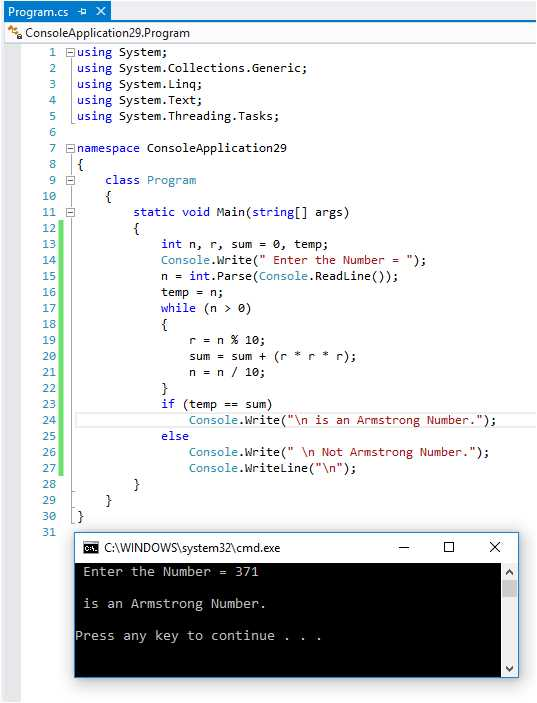What is an Armstrong Number in C# ?

Total Post:46

Points:324

570  View(s)
Ratings:
Rate this:

Like as -

371 = (3*3*3)+(7*7*7)+(1*1*1)

where:

(3*3*3)=27

(7*7*7)=343

(1*1*1)=1

So:

27+343+1=371

1.Re: What is an Armstrong Number in C# ?

This program for Practices ...

Armstrong Number Program...

Armstrong number is a number that is equal to the sum of cubes of its digits. For example 0, 1, 153, 370, 371 and 407 are the Armstrong numbers.

using System;

using System.Collections.Generic;
using System.Linq;
using System.Text;

namespace ConsoleApplication29
{
class Program
{
static void Main(string[] args)
{
int n, r, sum = 0, temp;
Console.Write(" Enter the Number = ");
temp = n;
while (n > 0)
{
r = n % 10;
sum = sum + (r * r * r);
n = n / 10;
}
if (temp == sum)
Console.Write("\n is an Armstrong Number.");
else
Console.Write(" \n Not Armstrong Number.");
Console.WriteLine("\n");
}
}
}Thank You....